HOME Electronics Directory Articles/ Tutorials eBooks About Us FORUM Links Contact Us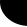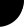"Verilog Tutorial"        by Harsha Perla Overview of Verilog HDL

If you can express working of a digital circuit and visualize the flow of data inside a IC, then learning any HDL or Hardware Description Language is very easy. This chapter is a overview of how Verilog code looks like.

This article will always be under construction.

Let us start with an AND gate. Here is the truth table: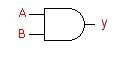A B Y 0 0 0 0 1 0 1 0 0 1 1 1

 ```/* A simple AND gate File: and.v */``` ```module andgate (a, b, y); input a, b; output y;``` `assign y = a & b;` `endmodule`

Now let us try to understand the code.

/* This is multi line
comment */

and           // this is single line comment, Comments are same as in C language.

In verilog, one circuit is represented by set of "modules". We can consider a module as a black box. With this assumption, if you draw a block diagram of the circuit with a set of signals connection each other, that is called top level design. Then go on writing modules for each black box, then design that black box with in the same way. This is how we are designing a circuit. You will understand this concept after studying some examples. A module may be one gate, one flip-flop, one register, one ALU one controller or one SOC. Go back to the example. Here, module is keyword, andgate is the name given to the module in this examples and a, b and y are the ports or connections to the module. Every modules and with the keyword endmodule.

In the beginning of a module, we have to declare all ports as input output or inout. By default, ports will have one pin or one bit.

Using 'assign' statement, we connected inputs and outputs via AND gate. A will be ANDed with B and will be connected to Y. Here, we are not going to store the values, and hence we did not declare any registers. By default, all the ports will be considered as wires. If we want the output to be latched, we have to declare it using the keyword 'reg'.

input a, b;
output y;
wire a, b, y;

Next we will write a testbench to test the gate that we have created. Testbench is another verilog code that creates a circuit involving the circuit to be tested. This code will send different inputs to the code under test and get the output and displays to check the accuracy.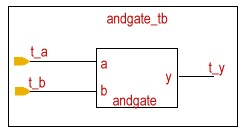/* testbench for AND gate File: and_tb.v */ module andgate_tb; wire t_y; reg t_a, t_b; andgate my_gate( .a(t_a), .b(t_b), .y(t_y) ); initial begin \$monitor(t_a, t_b, t_y); t_a = 1'b0; t_b = 1'b0; #5 t_a = 1'b0; t_b = 1'b1; #5 t_a = 1'b1; t_b = 1'b0; #5 t_a = 1'b1; t_b = 1'b1; end endmodule

Here we have created another module andgate_tb which will include the module andgate. We have to give values to input, so we need to store or latch the input data. So, t_a and t_b are declared as reg and t_y as wire fto get the outut value from the gate. Now,  we create an instance of andgate. i.e., we are placing the and gate that we have created previously inside this module. We can use two different notations to connect the ports.

We can write andgate my_gate(t_a, t_b, t_y);. This is called instanciating by order. Here, all the ports should be in the order as we declared in the module definition. If we write as given in the example, we can change the order. That is,
andgate my_gate( .a(t_a), .b(t_b), .y(t_y) ); is equal to
andgate my_gate( .b(t_b), .y(t_y), .a(t_a) );.
This is called instanciation by name. Like this, we can use any number of instances of same module in a design.

'a' in the andgate will be connected to t_a in the andgate_tb and so on. If some of the statements in our code is to be executed only in the beginning of the execution, we can write them using initial block. initial block executes only once and starts at time=0. Your program may have any number of initial blocks. initial block begins with begin and ends with end. Inside an initial block, statements will execute sequentially. In next chapter, we will learn more about flow of execution of a program.

\$monitor is a system task used to display the values in variable whenever value of one of its arguments changes. After that we will assign different values to t_a and t_b. Here 1'b0 indicates a low signal and 1'b1 represents a high signal. Meaning of this notation is, a 1 bit variable expressed in binary as 1. Similarly we can also indicates decimal, hex, octal numbers as follows.

 Integer Meaning Stored as 5'b00101 5 bit binary 00101 00101 8'b0 8 bit binary 00000000 00000000 8'b101 8 bit binary 00000101 00000101 8'd5 8 bit decimal 5 00000101 8'h9f 8 bit hex 9f 10011111 3'd1 3 bit decimal 1 001 4'bz 3 bit decimal z zzzz 4'bx1 binary xxx1 5'b11z binary 0011z 15 32 bit decimal 15 0....01111(32 bits) 'o5 32 bit octal 5 0....00101(32 bits)

Rules:

• 1: Active high bit

• 0: Active low bit

• z: high impedance

• x: Uncertain/ Don't care

• If not mentioned, length is 32 bit and data type is integer by default.

• If value is larger than the length, left most bits will be truncated

• If value is smaller,

• 0's are filled to the left if left most bit is 0 or 1

• 'z' are filled if left most bit is z

• 'x' are filled if left most bit is x

#5 indicates a delay of 5ns. To observe the changes in the output waveform, we need to include this delay. If we observe the output, we can notice that input changes in the intervals of 5ns and we will get corresponding ANDed output in t_y.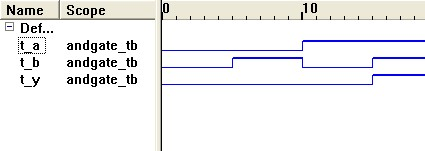Next: Verilog Primitives

Prev. : Table of Contents
TOC
Ch:  1. 2. 3. 4. 5. 6. 7. 8. 9. 10. 11. 12. 13.
Next: Verilog Primitives

 Home   |    About Us   |   Articles/ Tutorials   |   Downloads   |   Feedback   |   Links   |   eBooks   |   Privacy Policy Copyright © 2005-2007 electroSofts.com. webmaster@electroSofts.com